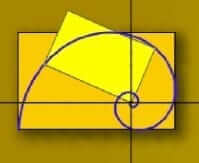\$89.00

Note: Geometry: A Guided Inquiry is out of print. However, you will get a free digital copy (PDF) when purchasing the Math Without Borders Home Study Companion.

This digital download provides a computer video series (from Math Without Borders) designed to accompany Paul A. Foerster’s Geometry: A Guided Inquiry. These video lessons introduce and cover each of the topics in Geometry: A Guided Inquiry and serve as an excellent companion to the text. This combination provides video instruction for each section of Foerster’s text, assigned problems for each day of work, and fully worked video solutions for assigned problems. The course makes frequent use of compass, protractor and ruler activities, data tables, guess and check methods, model-building, and other techniques of intuitive exploration in preparation for general solutions. This course also uses GeoGebra (Classic edition, Ver 5) as a calculation and graphing tool.

Description of Geometry: A Guided Inquiry from David Chandler (of Math Without Borders):

Geometry: A Guided Inquiry starts each chapter by posing a interesting geometric problem (puzzle), called the “Central Problem” for the chapter. Clusters of geometric facts are introduced, as needed, in the process of solving these problems. The usefulness and relevance of the new facts are therefore apparent from the moment they are first presented.

Each chapter has an open ended Projects section with problems that are extensions to the material in the Central section, sometimes carrying the discussion in new directions. The Project sections include some of the most interesting material in the text!”

• Chapter 1 The Shortest Path (i.e. distance between points and lines, perpendicular lines, reflection, triangle inequality, some properties of angles)
• Chapter 2 Tiling the Plane (i.e. polygons and angles)
• Chapter 3 Triangles (i.e. SSS, SAS, ASA congruence properties of triangles; proving theorems)
• Chapter 4 What is a Proof? (i.e. devising proofs and properties of quadrilaterals)
• Chapter 5 Constructions with Straightedge and Compass
• Chapter 7 Expressions and Equations Containing Two Variables
• Chapter 8 Area and Volume
• Chapter 9 The Pythagorean Theorem
• Chapter 10 Similar Figures
• Chapter 11 Perimeter, Area, and Volume of Similar Figures
• Chapter 12 Circles
• Chapter 13 Coordinates
• Chapter 14 Conic Sections